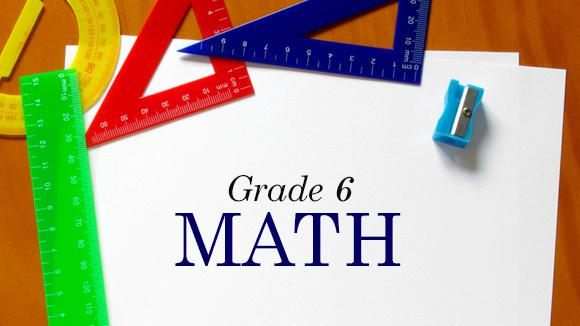COURSE DESCRIPTION6th grade math as an introductory middle level math course. Students will learn about statistical thinking and organizing data. Students also will learn about problem solving, exponents, and order of operations. Students will also learn basic Algebra and Geometry. By the end of this course students should also be able to perform operations using numbers other than whole numbers. This includes working with decimals, fractions, and mixed numbers. Students will learn to convert fractions to decimals and decimals to fractions. They should also understand how to multiply, divide, add, and subtract decimals, fractions, and mixed numbers.

CHAPTERSCh.1 – Geometry
Identify the Parts of a Solid
Parts of Geometric Figures
Constructing Geometric Figures
Areas of Polygons
Circumference and Area of a Circle
Similar Triangles
Circumference and Area
Identifying Parts of a Circle
Ordered Pairs
Areas on the Coordinate Plane
Areas of Irregular Polygons
Volume of a Rectangular Prism
Supplementary, Complementary and Vertical Angles
Geometry Test

Ch.2 – Number System
Operations on Mixed Numbers
Using Positive and Negative Numbers to Describe Quantities
Division of Fractions
Using Positive and Negative Numbers to Describe Quantities
Graphing Points
Exponents: Prime Factorization
Multiples and Least Common Multiples
Factors and Greatest Common Factor
Add, Subtract, Multiply and Divide Decimals
Multi-Digit Division
Identifying Factors
Comparing and Ordering Numbers in Different Forms
Absolute Value
Comparing and Ordering Numbers
Estimating Percents
Identifying Percents
Reciprocals
Operations on Mixed Numbers
Rational Numbers on a Number Line
Exponential Form
Coordinate Geometry
Multiply Multi-Digit Decimals
Fractions, Decimals and Percents
Multiply and Divide Fractions
Multiplying Decimals
Dividing with Decimals

Ch.3 – Expressions amd Equations
Describing Relationships Using Independent and Dependent Variables
Equivalent Expressions
Proving Expressions are Equivalent
Expressions Involving Exponents
Describing Relationships Using Independent and Dependent Variables
Proving Expressions are Equivalent
Equivalent Expressions
Expressions Involving Exponents
Expressions with Exponents
Translating Verbal Expressions into Inequalities
Solving Two-Step Equations
Writing Algebraic Expressions
Solving One-Step Equations
Translating Verbal Expressions
Evaluate Expressions with Rational Numbers
Order of Operations
Translating Mathematical Sentences
Expressions amd Equations Test

Ch.4 – Statistics and Probability
Statistical vs. Non-Statistical Questions
Summary Statistics
Median, Mode and Range
Predicting Experiment Results
Compare Summary Statistics
Dependent Events
Frequency Tables
Counting Principle
Interpreting Graphs
Venn Diagrams
Making Predictions Using Data
Statistics and Probability Test

﻿
QQ咨询